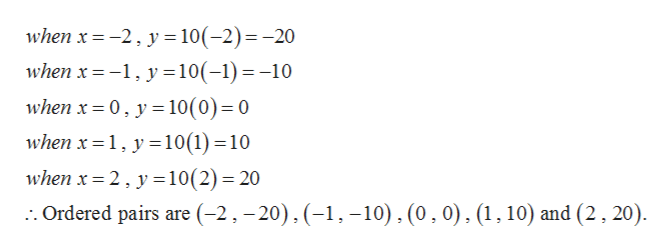Make a table listing ordered pairs that satisfy the equation. Then graph the equation. Determine the domain and​ range, and whether y is a function of x. y=10x

Question
Make a table listing ordered pairs that satisfy the equation. Then graph the equation. Determine the domain and​ range, and whether y is a function of x.

y=10x
Step 1

Given,

Step 2

Now computing the values of y for each values of x, we gethelp_outlineImage Transcriptionclosewhen x-2y 10(-2)=-20 when x 10(-1)-10 when x 0, y 10(0) 0 when x 1,y10(1)10 when x 2y 10(2) 20 :. Ordered pairs are (-2, -20), (-1 10), (0,0), (1,10) and (2, 20) fullscreen
Step 3

The graph is,

&n...

Want to see the full answer?

See Solution

Want to see this answer and more?

Our solutions are written by experts, many with advanced degrees, and available 24/7

See Solution
Tagged in

Algebra